search: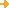advanced search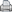printer-friendly version

Title: A New View of Statistics
Url: http://www.sportsci.org/resource/stats/index.html
Creator: Hopkins, Will G.
Publisher: Hopkins, Will G.
 Description: This online resource is intended to help students understand concepts from probability and statistics and covers many topics from introductory to advanced. You can follow the progression of the text, or you can click on a topic on the left. Key words include: alpha reliability, analysis of covariance (ANCOVA), analysis of variance (ANOVA), Bayesian analysis, bias, binomial regression, Bonferroni adjustment, bootstrapping, Categorical modeling, central limit theorem; Chi-squared test, clinical significance, cluster analysis, coefficient of variation, confidence intervals, contingency table, controlled trial, confounders, correlation, dimension reduction, discriminant function analysis, frequency, Normal, Poisson, probability Distributions, effect, error, factor analysis, goodness of fit, heteroscedasticity, hypothesis testing, independence, interactions, Kappa coefficient, Latin squares, least squares means, likert scales, linear regression, logistic regression, multivariate ANOVA (MANOVA), mixed modeling, multiple linear regression, nonparametric models, odds ratio, P values, path analysis, percentiles, polynomial regression, power, PRESS, probability, relative frequency; repeated measures; sample size, sampling, sensitivity; stepwise regression, structural equation modeling, T test, transformation, and validity. This is a large, and fairly comprehensive, collection of statistical concepts.
LC Classification: Science -- Mathematics -- Analysis -- Functional analysis -- Theory of distributions
Science -- Mathematics -- Mathematical statistics -- Analysis of variance and covariance. Experimental design
Science -- Mathematics -- Mathematical statistics -- Study and teaching. Research
Science -- Mathematics -- Probabilities -- Study and teaching. Research
GEM Subject: Mathematics -- Statistics
Mathematics -- Probability
Key Concept: Mathematics -- Bayes’ formula
Mathematics -- Binomial distribution
Mathematics -- Chi square
Mathematics -- Confidence intervals
Mathematics -- Frequency distributions
Mathematics -- Histograms
Mathematics -- Hypothesis testing
Mathematics -- Normal distribution
Mathematics -- Null hypothesis
Mathematics -- Probability
Mathematics -- Standard deviation
Mathematics -- Statistics
Mathematics -- t-distribution
Mathematics -- Tests of variance
Mathematics -- Variation
Mathematics -- z-scores
Date Issued: 2008
Resource Type: Collection
Reference Material
Instructional Material -- Student Guide
Instructional Material -- Interactive Simulation
Instructional Material -- Instructor Guide/Manual
Format: Document -- HTML
Audience: Educator
Learner
Education Level: Higher Education
Higher Education -- Undergraduate (Lower Division)
Higher Education -- Undergraduate (Upper Division)
Higher Education -- Technical Education (Upper Division)
Higher Education -- Technical Education (Lower Division)
Vocational/Professional Development Education
Language: English
Rights: Will G. Hopkins
Access Rights: Free access
 ScreenshotFull Image
Source: CAUSEweb
Full Record Views: 22
Resource URL Clicks: 11
Cumulative Rating: NOT YET RATED
 Report a Problem with this Resource Record

(no comments available yet for this resource)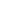forget your password?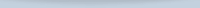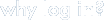Save, organize, and share resources that you find.

Subscribe to bulletins

It's easy, fast, and FREE!get your own loginAMSER =
FREE ONLINE
RESOURCES
for the
CLASSROOM